# Building Java Programs Chapter 12 Recursion Recursion recursion

• Slides: 62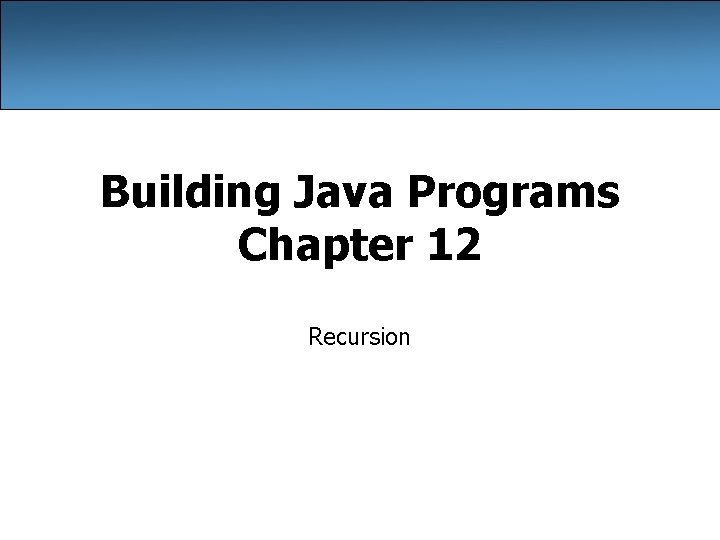Building Java Programs Chapter 12 Recursion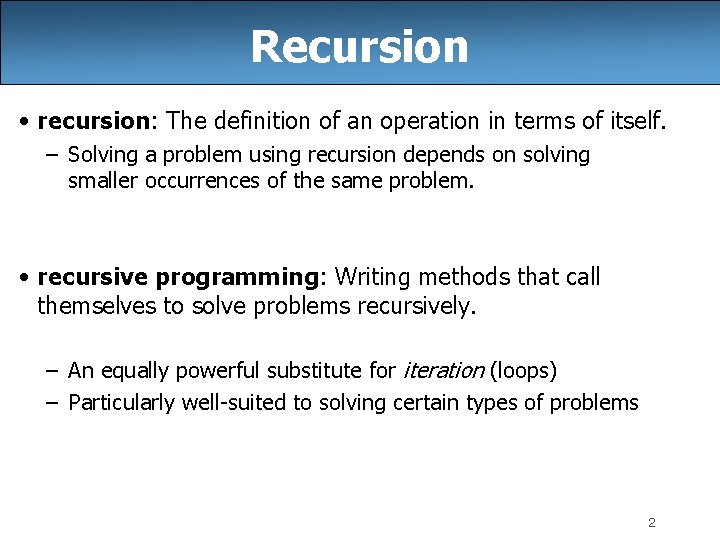Recursion • recursion: The definition of an operation in terms of itself. – Solving a problem using recursion depends on solving smaller occurrences of the same problem. • recursive programming: Writing methods that call themselves to solve problems recursively. – An equally powerful substitute for iteration (loops) – Particularly well-suited to solving certain types of problems 2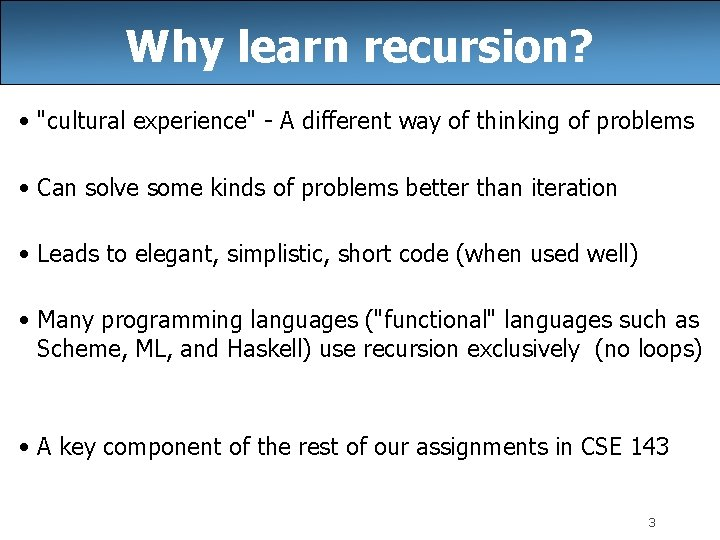Why learn recursion? • "cultural experience" - A different way of thinking of problems • Can solve some kinds of problems better than iteration • Leads to elegant, simplistic, short code (when used well) • Many programming languages ("functional" languages such as Scheme, ML, and Haskell) use recursion exclusively (no loops) • A key component of the rest of our assignments in CSE 143 3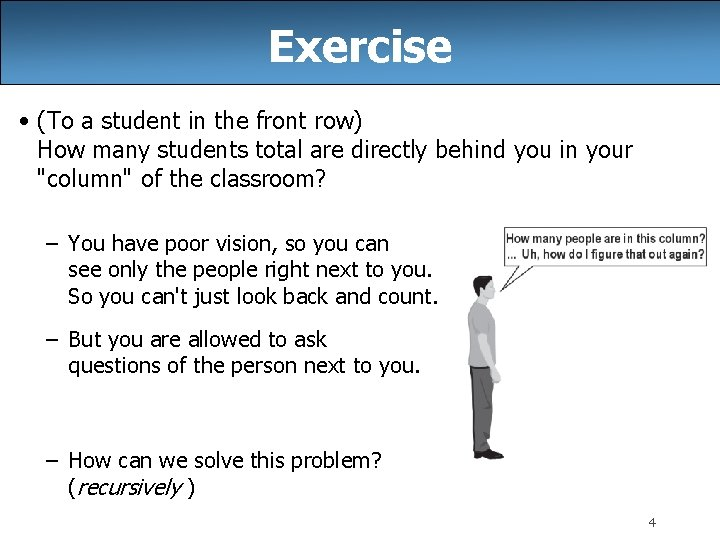Exercise • (To a student in the front row) How many students total are directly behind you in your "column" of the classroom? – You have poor vision, so you can see only the people right next to you. So you can't just look back and count. – But you are allowed to ask questions of the person next to you. – How can we solve this problem? (recursively ) 4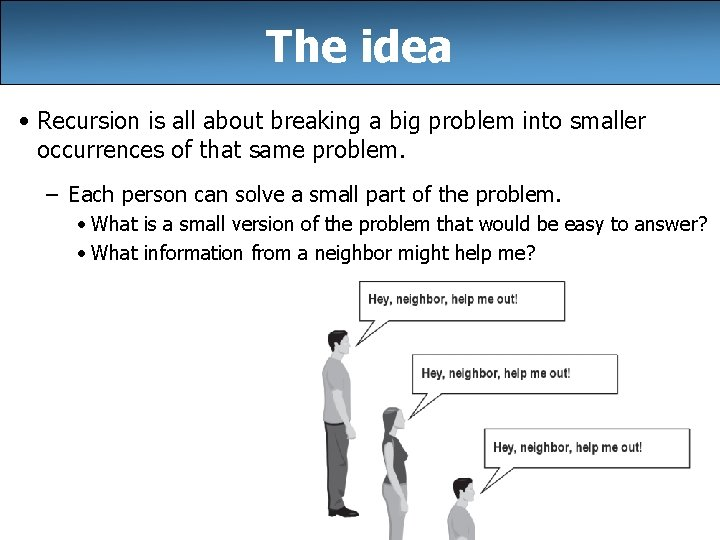The idea • Recursion is all about breaking a big problem into smaller occurrences of that same problem. – Each person can solve a small part of the problem. • What is a small version of the problem that would be easy to answer? • What information from a neighbor might help me? 5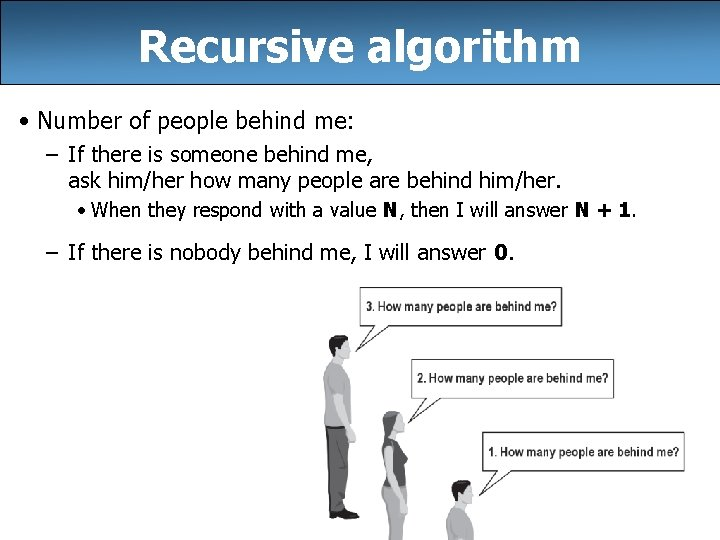Recursive algorithm • Number of people behind me: – If there is someone behind me, ask him/her how many people are behind him/her. • When they respond with a value N, then I will answer N + 1. – If there is nobody behind me, I will answer 0. 6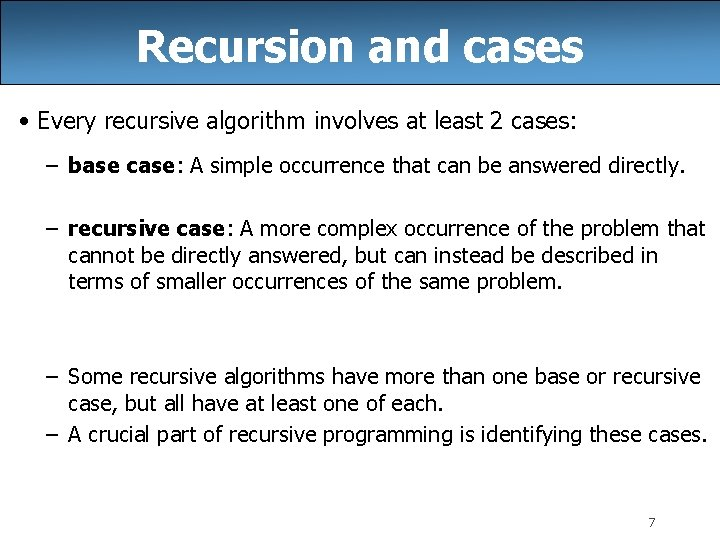Recursion and cases • Every recursive algorithm involves at least 2 cases: – base case: A simple occurrence that can be answered directly. – recursive case: A more complex occurrence of the problem that cannot be directly answered, but can instead be described in terms of smaller occurrences of the same problem. – Some recursive algorithms have more than one base or recursive case, but all have at least one of each. – A crucial part of recursive programming is identifying these cases. 7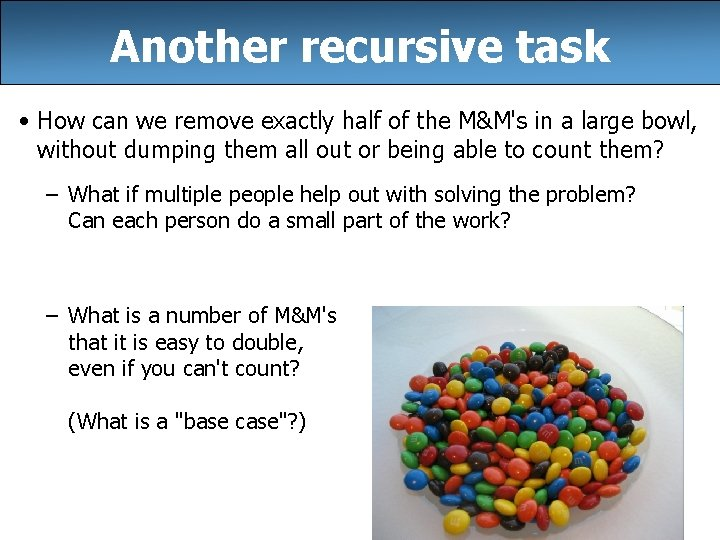Another recursive task • How can we remove exactly half of the M&M's in a large bowl, without dumping them all out or being able to count them? – What if multiple people help out with solving the problem? Can each person do a small part of the work? – What is a number of M&M's that it is easy to double, even if you can't count? (What is a "base case"? ) 8Recursion in Java • Consider the following method to print a line of * characters: // Prints a line containing the given number of stars. // Precondition: n >= 0 public static void print. Stars(int n) { for (int i = 0; i < n; i++) { System. out. print("*"); } System. out. println(); // end the line of output } • Write a recursive version of this method (that calls itself). – Solve the problem without using any loops. – Hint: Your solution should print just one star at a time. 9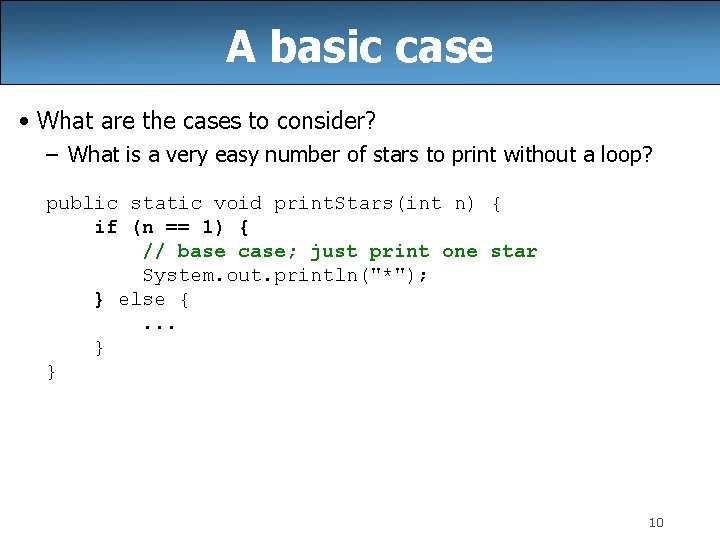A basic case • What are the cases to consider? – What is a very easy number of stars to print without a loop? public static void print. Stars(int n) { if (n == 1) { // base case; just print one star System. out. println("*"); } else {. . . } } 10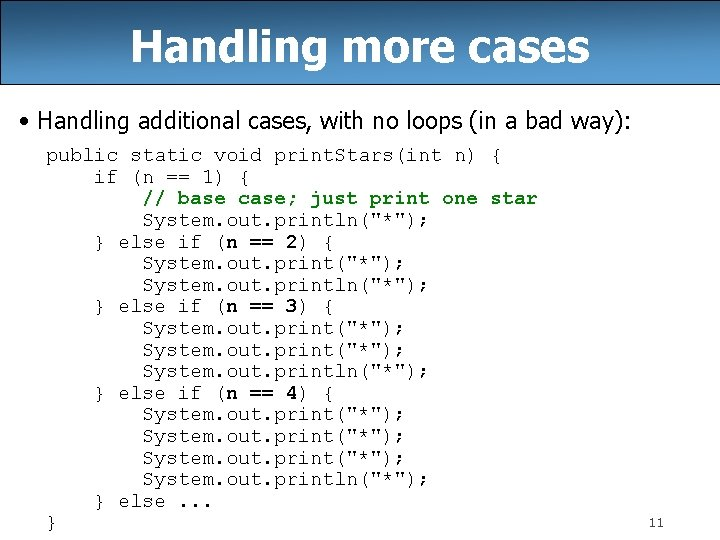Handling more cases • Handling additional cases, with no loops (in a bad way): public static void print. Stars(int n) { if (n == 1) { // base case; just print one star System. out. println("*"); } else if (n == 2) { System. out. print("*"); System. out. println("*"); } else if (n == 3) { System. out. print("*"); System. out. println("*"); } else if (n == 4) { System. out. print("*"); System. out. println("*"); } else. . . } 11Handling more cases 2 • Taking advantage of the repeated pattern (somewhat better): public static void print. Stars(int n) { if (n == 1) { // base case; just print one star System. out. println("*"); } else if (n == 2) { System. out. print("*"); print. Stars(1); // prints "*" } else if (n == 3) { System. out. print("*"); print. Stars(2); // prints "**" } else if (n == 4) { System. out. print("*"); print. Stars(3); // prints "***" } else. . . } 12Using recursion properly • Condensing the recursive cases into a single case: public static void print. Stars(int n) { if (n == 1) { // base case; just print one star System. out. println("*"); } else { // recursive case; print one more star System. out. print("*"); print. Stars(n - 1); } } 13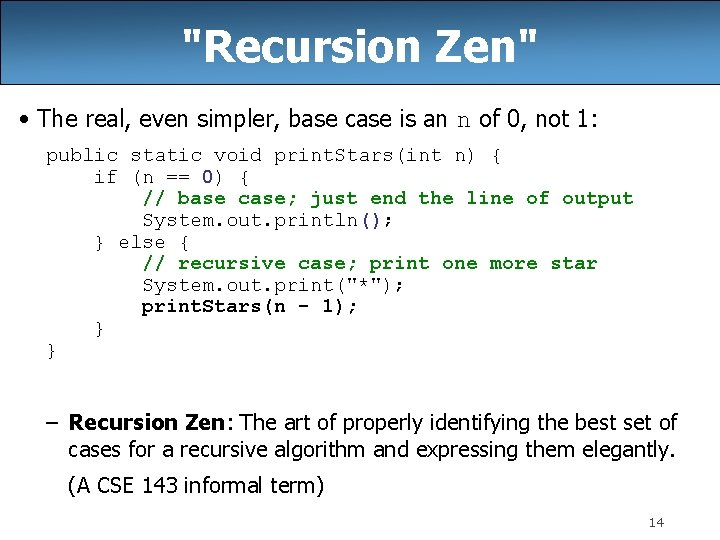"Recursion Zen" • The real, even simpler, base case is an n of 0, not 1: public static void print. Stars(int n) { if (n == 0) { // base case; just end the line of output System. out. println(); } else { // recursive case; print one more star System. out. print("*"); print. Stars(n - 1); } } – Recursion Zen: The art of properly identifying the best set of cases for a recursive algorithm and expressing them elegantly. (A CSE 143 informal term) 14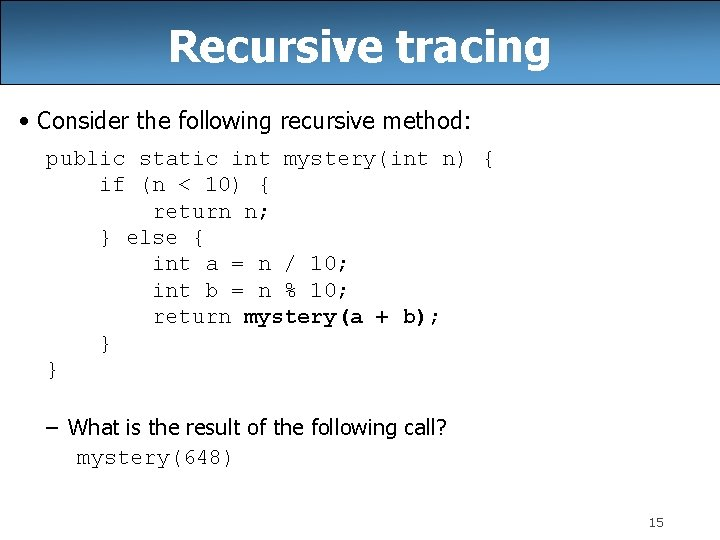Recursive tracing • Consider the following recursive method: public static int mystery(int n) { if (n < 10) { return n; } else { int a = n / 10; int b = n % 10; return mystery(a + b); } } – What is the result of the following call? mystery(648) 15A recursive trace mystery(648): § int a = 648 / 10; § int b = 648 % 10; § return mystery(a + b); mystery(72): § int a = 72 / 10; § int b = 72 % 10; § return mystery(a + b); // 64 // 8 // mystery(72) // 7 // 2 // mystery(9): § return 9; 16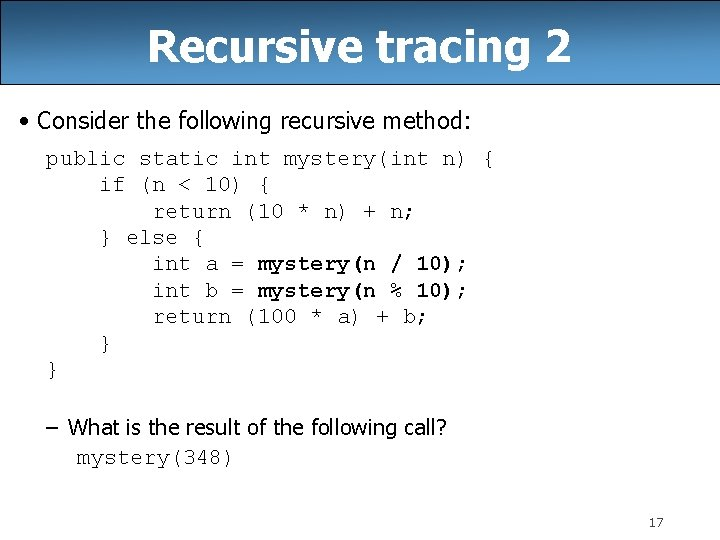Recursive tracing 2 • Consider the following recursive method: public static int mystery(int n) { if (n < 10) { return (10 * n) + n; } else { int a = mystery(n / 10); int b = mystery(n % 10); return (100 * a) + b; } } – What is the result of the following call? mystery(348) 17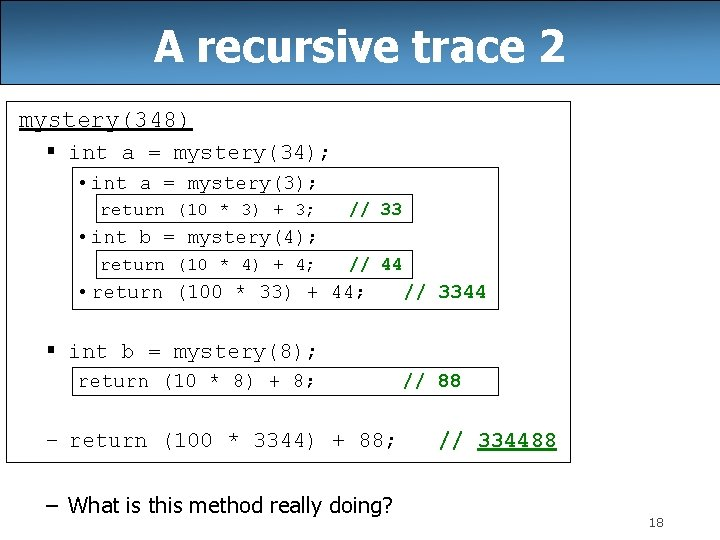A recursive trace 2 mystery(348) § int a = mystery(34); • int a = mystery(3); return (10 * 3) + 3; // 33 • int b = mystery(4); return (10 * 4) + 4; // 44 • return (100 * 33) + 44; // 3344 § int b = mystery(8); return (10 * 8) + 8; – return (100 * 3344) + 88; – What is this method really doing? // 88 // 334488 18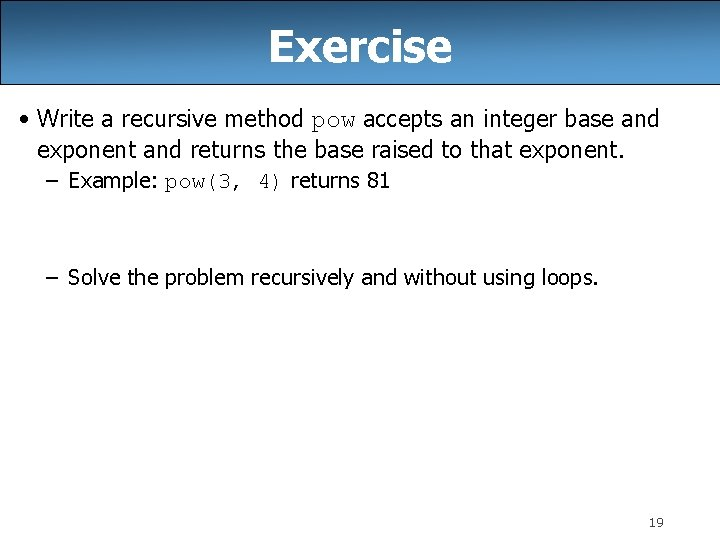Exercise • Write a recursive method pow accepts an integer base and exponent and returns the base raised to that exponent. – Example: pow(3, 4) returns 81 – Solve the problem recursively and without using loops. 19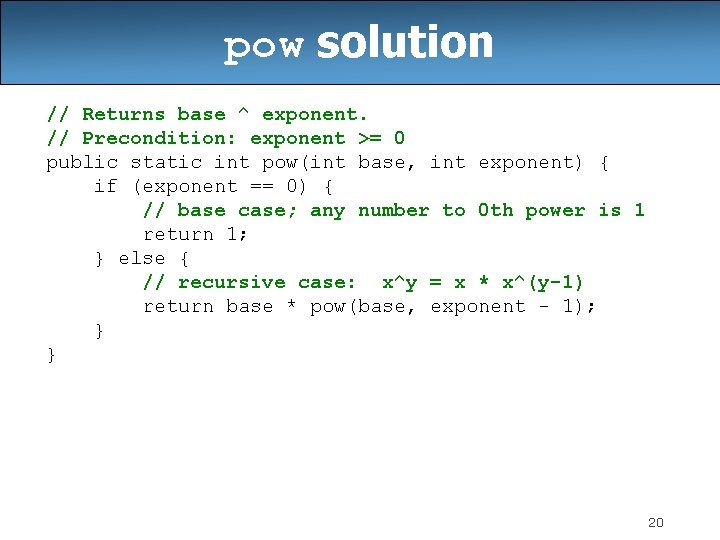pow solution // Returns base ^ exponent. // Precondition: exponent >= 0 public static int pow(int base, int exponent) { if (exponent == 0) { // base case; any number to 0 th power is 1 return 1; } else { // recursive case: x^y = x * x^(y-1) return base * pow(base, exponent - 1); } } 20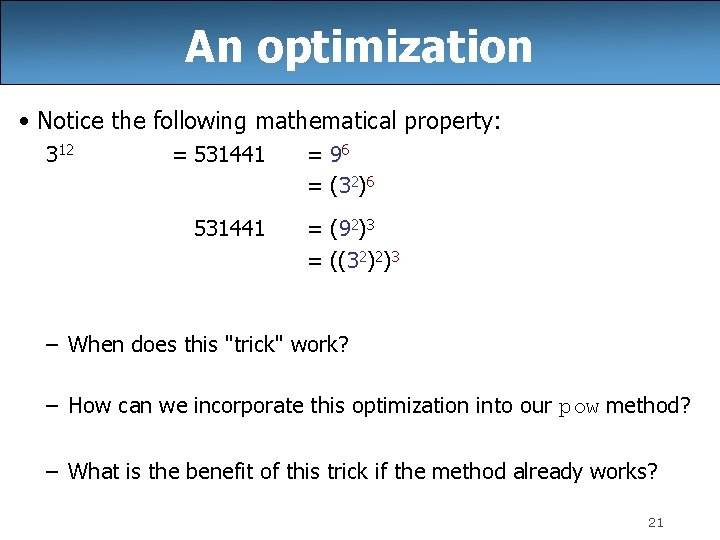An optimization • Notice the following mathematical property: 312 = 531441 = 96 = (32)6 = (92)3 = ((32)2)3 – When does this "trick" work? – How can we incorporate this optimization into our pow method? – What is the benefit of this trick if the method already works? 21pow solution 2 // Returns base ^ exponent. // Precondition: exponent >= 0 public static int pow(int base, int exponent) { if (exponent == 0) { // base case; any number to 0 th power is 1 return 1; } else if (exponent % 2 == 0) { // recursive case 1: x^y = (x^2)^(y/2) return pow(base * base, exponent / 2); } else { // recursive case 2: x^y = x * x^(y-1) return base * pow(base, exponent - 1); } } 22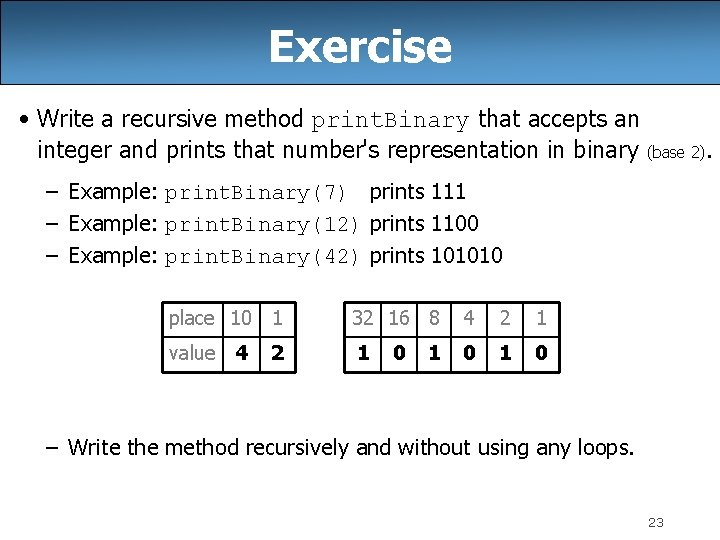Exercise • Write a recursive method print. Binary that accepts an integer and prints that number's representation in binary (base 2). – Example: print. Binary(7) prints 111 – Example: print. Binary(12) prints 1100 – Example: print. Binary(42) prints 101010 place 10 1 32 16 8 4 2 1 value 4 1 0 2 0 1 – Write the method recursively and without using any loops. 23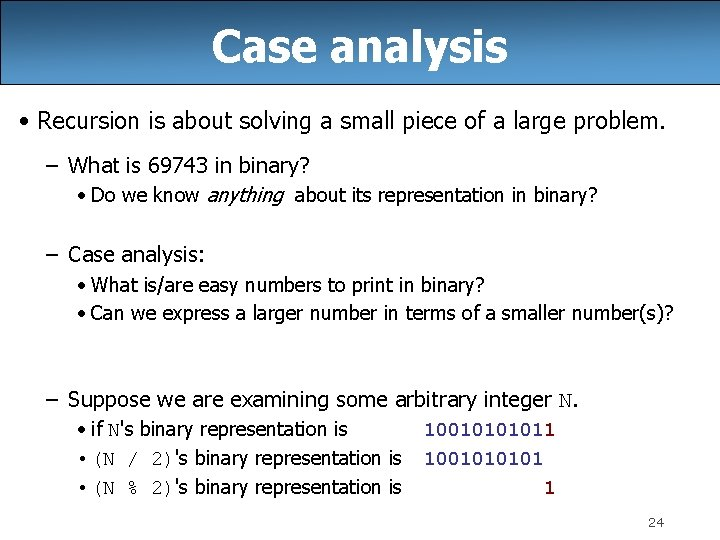Case analysis • Recursion is about solving a small piece of a large problem. – What is 69743 in binary? • Do we know anything about its representation in binary? – Case analysis: • What is/are easy numbers to print in binary? • Can we express a larger number in terms of a smaller number(s)? – Suppose we are examining some arbitrary integer N. • if N's binary representation is • (N / 2)'s binary representation is • (N % 2)'s binary representation is 1001011 100101 1 24print. Binary solution // Prints the given integer's binary representation. // Precondition: n >= 0 public static void print. Binary(int n) { if (n < 2) { // base case; same as base 10 System. out. println(n); } else { // recursive case; break number apart print. Binary(n / 2); print. Binary(n % 2); } } – Can we eliminate the precondition and deal with negatives? 25print. Binary solution 2 // Prints the given integer's binary representation. public static void print. Binary(int n) { if (n < 0) { // recursive case for negative numbers System. out. print("-"); print. Binary(-n); } else if (n < 2) { // base case; same as base 10 System. out. println(n); } else { // recursive case; break number apart print. Binary(n / 2); print. Binary(n % 2); } } 26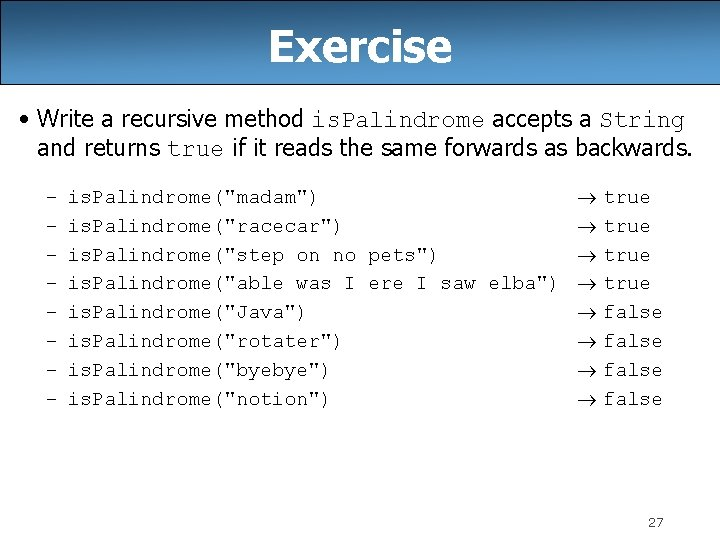Exercise • Write a recursive method is. Palindrome accepts a String and returns true if it reads the same forwards as backwards. – – – – is. Palindrome("madam") true is. Palindrome("racecar") true is. Palindrome("step on no pets") true is. Palindrome("able was I ere I saw elba") true is. Palindrome("Java") false is. Palindrome("rotater") false is. Palindrome("byebye") false is. Palindrome("notion") false 27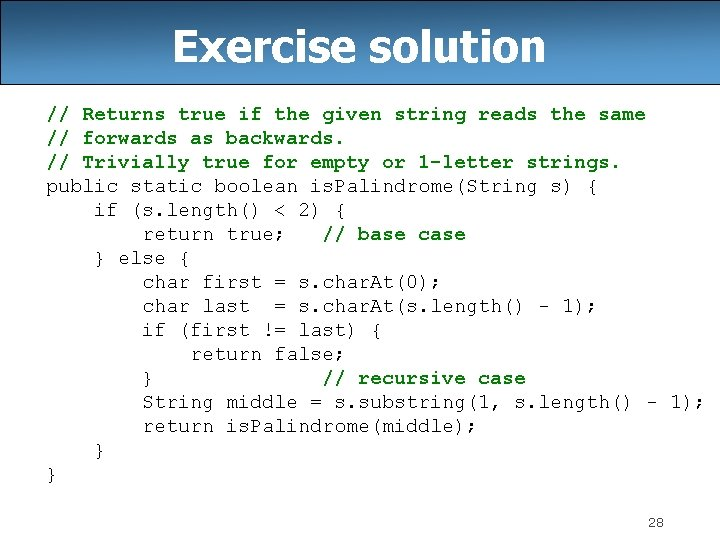Exercise solution // Returns true if the given string reads the same // forwards as backwards. // Trivially true for empty or 1 -letter strings. public static boolean is. Palindrome(String s) { if (s. length() < 2) { return true; // base case } else { char first = s. char. At(0); char last = s. char. At(s. length() - 1); if (first != last) { return false; } // recursive case String middle = s. substring(1, s. length() - 1); return is. Palindrome(middle); } } 28Exercise solution 2 // Returns true if the given string reads the same // forwards as backwards. // Trivially true for empty or 1 -letter strings. public static boolean is. Palindrome(String s) { if (s. length() < 2) { return true; // base case } else { return s. char. At(0) == s. char. At(s. length() - 1) && is. Palindrome(s. substring(1, s. length() - 1)); } } 29Exercise • Write a recursive method reverse. Lines that accepts a file Scanner and prints the lines of the file in reverse order. – Example input file: Roses are red, Violets are blue. All my base Are belong to you. Expected console output: Are belong to you. All my base Violets are blue. Roses are red, – What are the cases to consider? • How can we solve a small part of the problem at a time? • What is a file that is very easy to reverse? 30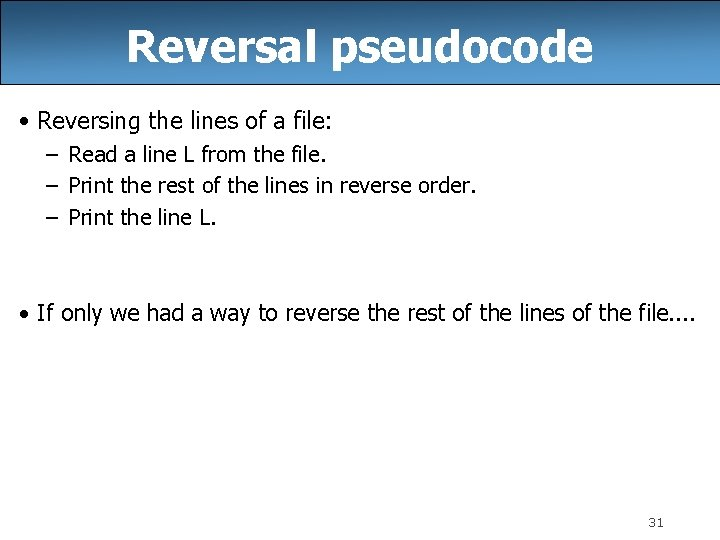Reversal pseudocode • Reversing the lines of a file: – Read a line L from the file. – Print the rest of the lines in reverse order. – Print the line L. • If only we had a way to reverse the rest of the lines of the file. . 31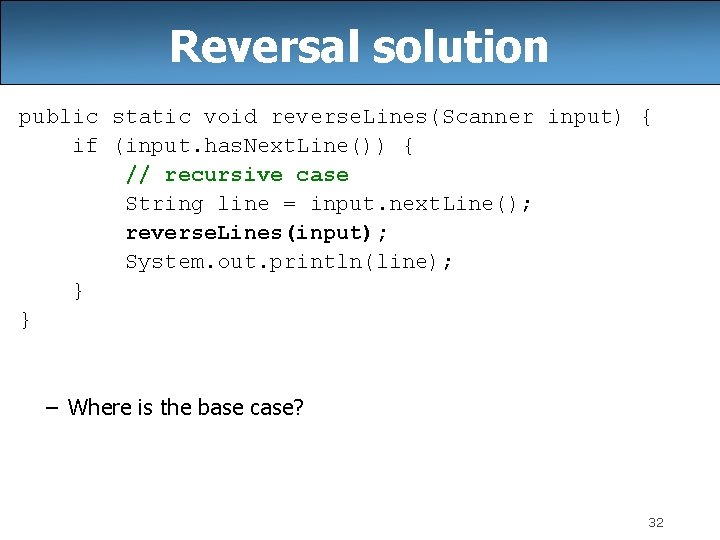Reversal solution public static void reverse. Lines(Scanner input) { if (input. has. Next. Line()) { // recursive case String line = input. next. Line(); reverse. Lines(input); System. out. println(line); } } – Where is the base case? 32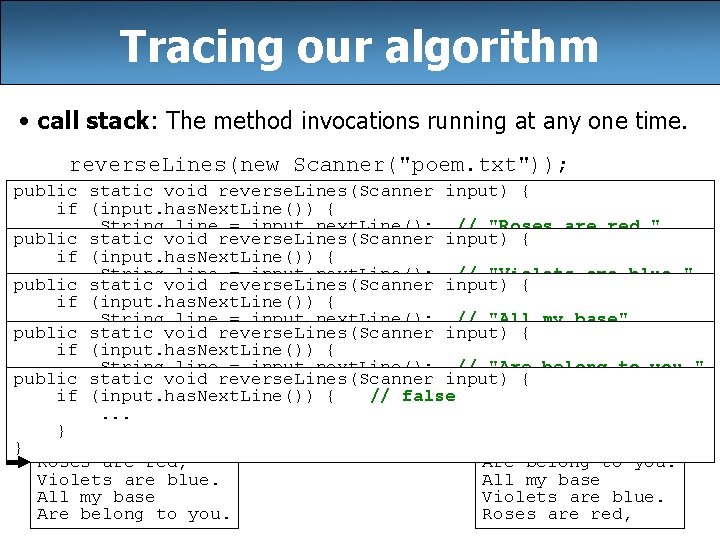Tracing our algorithm • call stack: The method invocations running at any one time. reverse. Lines(new Scanner("poem. txt")); public static void reverse. Lines(Scanner input) { if (input. has. Next. Line()) { String line = input. next. Line(); // "Roses are red, " public static void reverse. Lines(Scanner input) { reverse. Lines(input); if (input. has. Next. Line()) { System. out. println(line); String line = input. next. Line(); // "Violets are blue. " } static public void reverse. Lines(Scanner input) { reverse. Lines(input); } if (input. has. Next. Line()) { System. out. println(line); String line = input. next. Line(); // "All my base" } static public void reverse. Lines(Scanner input) { reverse. Lines(input); } if (input. has. Next. Line()) { System. out. println(line); String line = input. next. Line(); // "Are belong to you. " } static public void reverse. Lines(Scanner input) { reverse. Lines(input); } if (input. has. Next. Line()) { // false System. out. println(line); . . . } } file: } input output: } Roses are red, Are belong to you. Violets are blue. All my base Violets are blue. Are belong to you. Roses are red, 33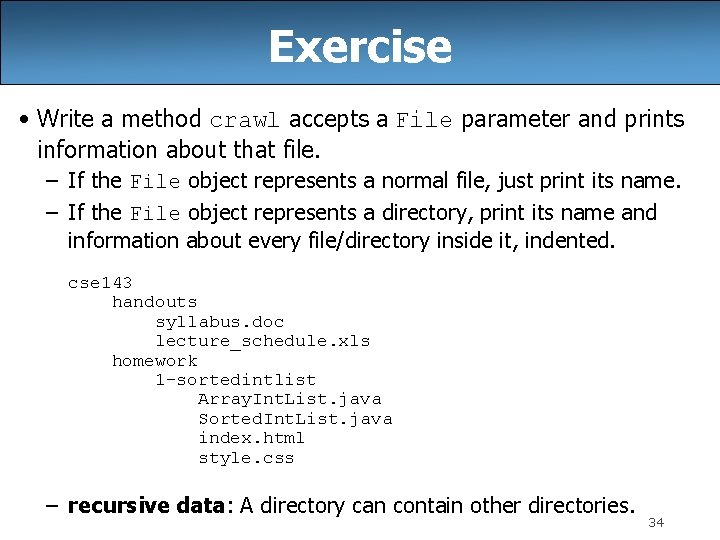Exercise • Write a method crawl accepts a File parameter and prints information about that file. – If the File object represents a normal file, just print its name. – If the File object represents a directory, print its name and information about every file/directory inside it, indented. cse 143 handouts syllabus. doc lecture_schedule. xls homework 1 -sortedintlist Array. Int. List. java Sorted. Int. List. java index. html style. css – recursive data: A directory can contain other directories. 34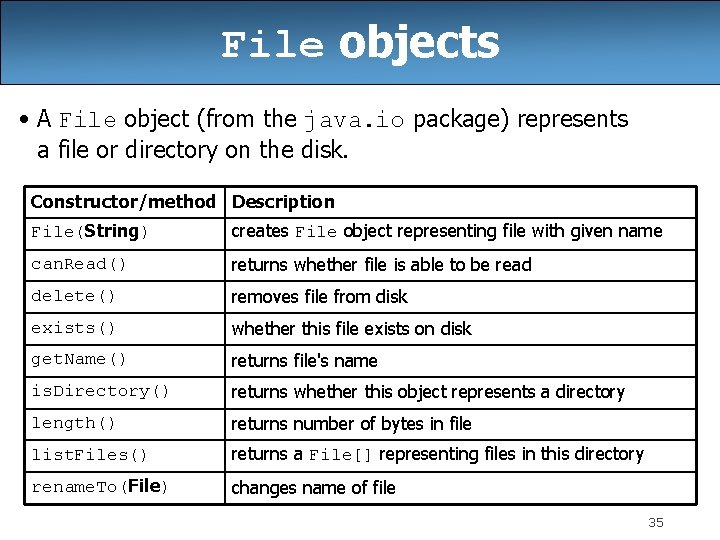File objects • A File object (from the java. io package) represents a file or directory on the disk. Constructor/method Description File(String) creates File object representing file with given name can. Read() returns whether file is able to be read delete() removes file from disk exists() whether this file exists on disk get. Name() returns file's name is. Directory() returns whether this object represents a directory length() returns number of bytes in file list. Files() returns a File[] representing files in this directory rename. To(File) changes name of file 35Public/private pairs • We cannot vary the indentation without an extra parameter: public static void crawl(File f, String indent) { • Often the parameters we need for our recursion do not match those the client will want to pass. In these cases, we instead write a pair of methods: 1) a public, non-recursive one with the parameters the client wants 2) a private, recursive one with the parameters we really need 36Exercise solution 2 // Prints information about this file, // and (if it is a directory) any files inside it. public static void crawl(File f) { crawl(f, ""); // call private recursive helper } // Recursive helper to implement crawl/indent behavior. private static void crawl(File f, String indent) { System. out. println(indent + f. get. Name()); if (f. is. Directory()) { // recursive case; print contained files/dirs for (File sub. File : f. list. Files()) { crawl(sub. File, indent + " "); } } } 37Recursive Backtracking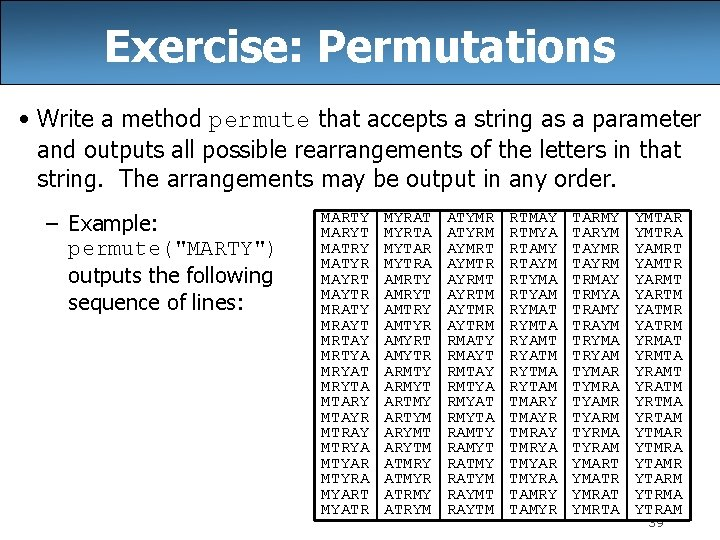Exercise: Permutations • Write a method permute that accepts a string as a parameter and outputs all possible rearrangements of the letters in that string. The arrangements may be output in any order. – Example: permute("MARTY") outputs the following sequence of lines: MARTY MARYT MATRY MATYR MAYRT MAYTR MRATY MRAYT MRTAY MRTYA MRYAT MRYTA MTARY MTAYR MTRAY MTRYA MTYAR MTYRA MYART MYATR MYRAT MYRTA MYTAR MYTRA AMRTY AMRYT AMTRY AMTYR AMYRT AMYTR ARMTY ARMYT ARTMY ARTYM ARYMT ARYTM ATMRY ATMYR ATRMY ATRYM ATYMR ATYRM AYMRT AYMTR AYRMT AYRTM AYTMR AYTRM RMATY RMAYT RMTAY RMTYA RMYAT RMYTA RAMTY RAMYT RATMY RATYM RAYMT RAYTM RTMAY RTMYA RTAMY RTAYM RTYMA RTYAM RYMAT RYMTA RYAMT RYATM RYTMA RYTAM TMARY TMAYR TMRAY TMRYA TMYAR TMYRA TAMRY TAMYR TARMY TARYM TAYMR TAYRM TRMAY TRMYA TRAMY TRAYM TRYMA TRYAM TYMAR TYMRA TYAMR TYARM TYRMA TYRAM YMART YMATR YMRAT YMRTA YMTAR YMTRA YAMRT YAMTR YARMT YARTM YATMR YATRM YRMAT YRMTA YRAMT YRATM YRTMA YRTAM YTMAR YTMRA YTAMR YTARM YTRMA YTRAM 39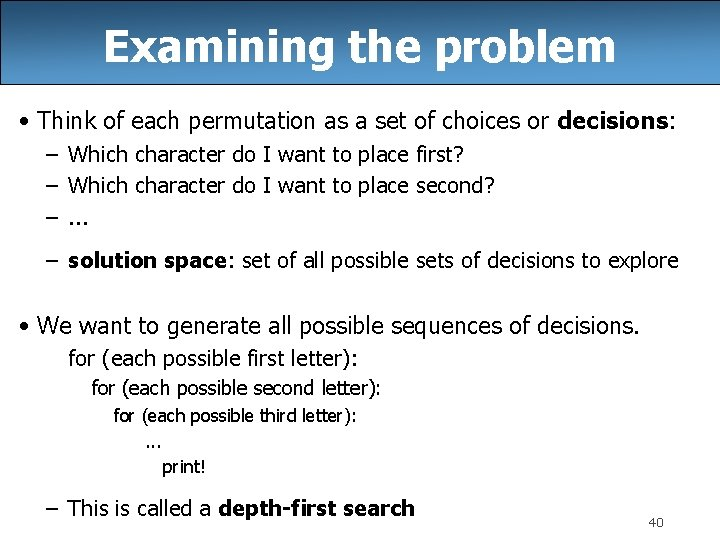Examining the problem • Think of each permutation as a set of choices or decisions: – Which character do I want to place first? – Which character do I want to place second? –. . . – solution space: set of all possible sets of decisions to explore • We want to generate all possible sequences of decisions. for (each possible first letter): for (each possible second letter): for (each possible third letter): . . . print! – This is called a depth-first search 40Decision trees chosen available MARTY A MRTY. . . M ARTY MAR TY MR ATY. . . MAT RY. . . MT ARY. . . MAY RT MY ART. . . MYA RT. . . MART Y MARY T MAYR T MAYT R MARTY MARYT MAYRT MAYTR 41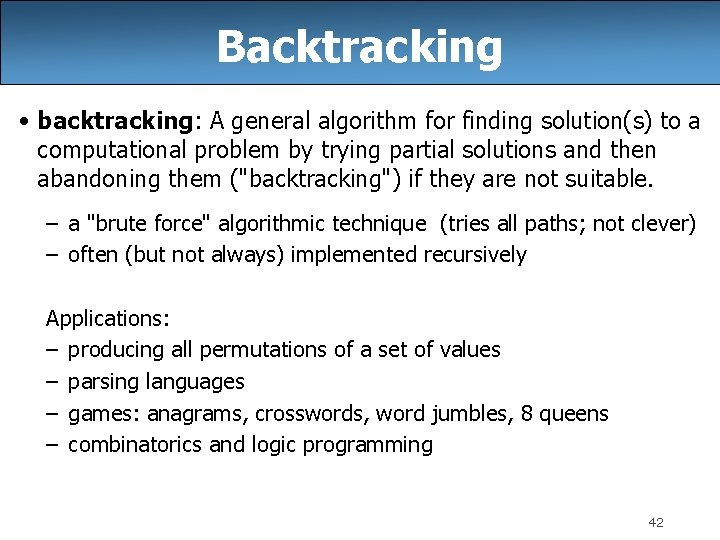Backtracking • backtracking: A general algorithm for finding solution(s) to a computational problem by trying partial solutions and then abandoning them ("backtracking") if they are not suitable. – a "brute force" algorithmic technique (tries all paths; not clever) – often (but not always) implemented recursively Applications: – producing all permutations of a set of values – parsing languages – games: anagrams, crosswords, word jumbles, 8 queens – combinatorics and logic programming 42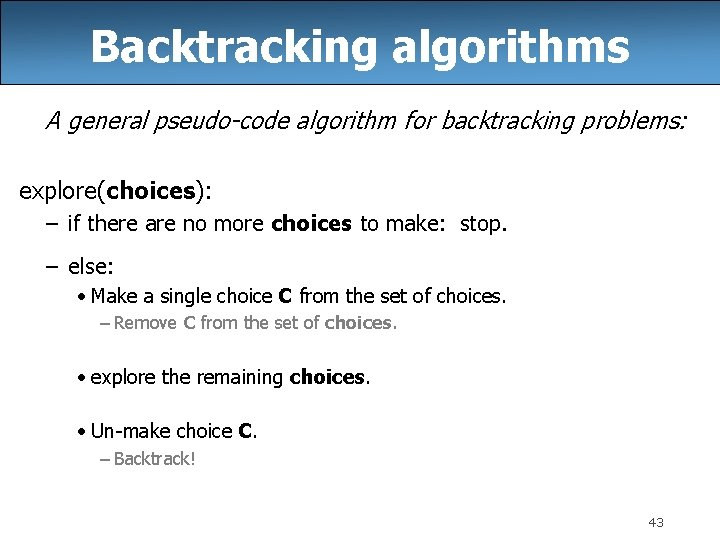Backtracking algorithms A general pseudo-code algorithm for backtracking problems: explore(choices): – if there are no more choices to make: stop. – else: • Make a single choice C from the set of choices. – Remove C from the set of choices. • explore the remaining choices. • Un-make choice C. – Backtrack! 43Backtracking strategies • When solving a backtracking problem, ask these questions: – What are the "choices" in this problem? • What is the "base case"? (How do I know when I'm out of choices? ) – How do I "make" a choice? • Do I need to create additional variables to remember my choices? • Do I need to modify the values of existing variables? – How do I explore the rest of the choices? • Do I need to remove the made choice from the list of choices? – Once I'm done exploring the rest, what should I do? – How do I "un-make" a choice? 44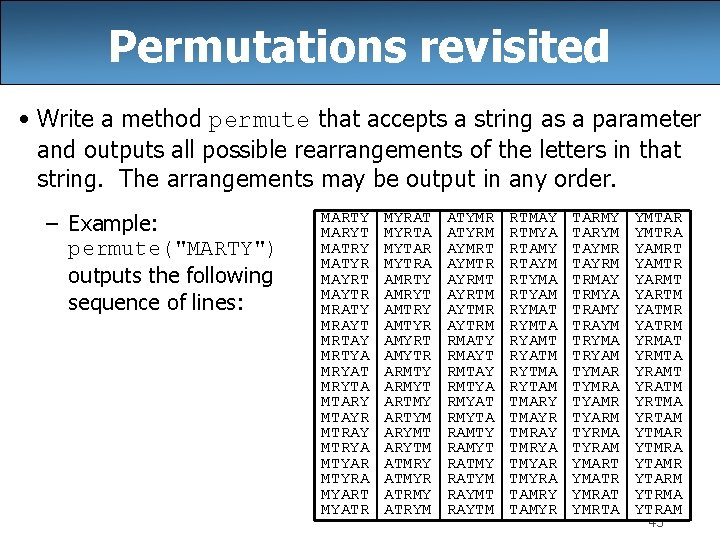Permutations revisited • Write a method permute that accepts a string as a parameter and outputs all possible rearrangements of the letters in that string. The arrangements may be output in any order. – Example: permute("MARTY") outputs the following sequence of lines: MARTY MARYT MATRY MATYR MAYRT MAYTR MRATY MRAYT MRTAY MRTYA MRYAT MRYTA MTARY MTAYR MTRAY MTRYA MTYAR MTYRA MYART MYATR MYRAT MYRTA MYTAR MYTRA AMRTY AMRYT AMTRY AMTYR AMYRT AMYTR ARMTY ARMYT ARTMY ARTYM ARYMT ARYTM ATMRY ATMYR ATRMY ATRYM ATYMR ATYRM AYMRT AYMTR AYRMT AYRTM AYTMR AYTRM RMATY RMAYT RMTAY RMTYA RMYAT RMYTA RAMTY RAMYT RATMY RATYM RAYMT RAYTM RTMAY RTMYA RTAMY RTAYM RTYMA RTYAM RYMAT RYMTA RYAMT RYATM RYTMA RYTAM TMARY TMAYR TMRAY TMRYA TMYAR TMYRA TAMRY TAMYR TARMY TARYM TAYMR TAYRM TRMAY TRMYA TRAMY TRAYM TRYMA TRYAM TYMAR TYMRA TYAMR TYARM TYRMA TYRAM YMART YMATR YMRAT YMRTA YMTAR YMTRA YAMRT YAMTR YARMT YARTM YATMR YATRM YRMAT YRMTA YRAMT YRATM YRTMA YRTAM YTMAR YTMRA YTAMR YTARM YTRMA YTRAM 45Exercise solution // Outputs all permutations of the given string. public static void permute(String s) { permute(s, ""); } private static void permute(String s, String chosen) { if (s. length() == 0) { // base case: no choices left to be made System. out. println(chosen); } else { // recursive case: choose each possible next letter for (int i = 0; i < s. length(); i++) { char c = s. char. At(i); // choose s = s. substring(0, i) + s. substring(i + 1); chosen += c; permute(s, chosen); } } } // explore s = s. substring(0, i) + c + s. substring(i + 1); chosen = chosen. substring(0, chosen. length() - 1); // un-choose 46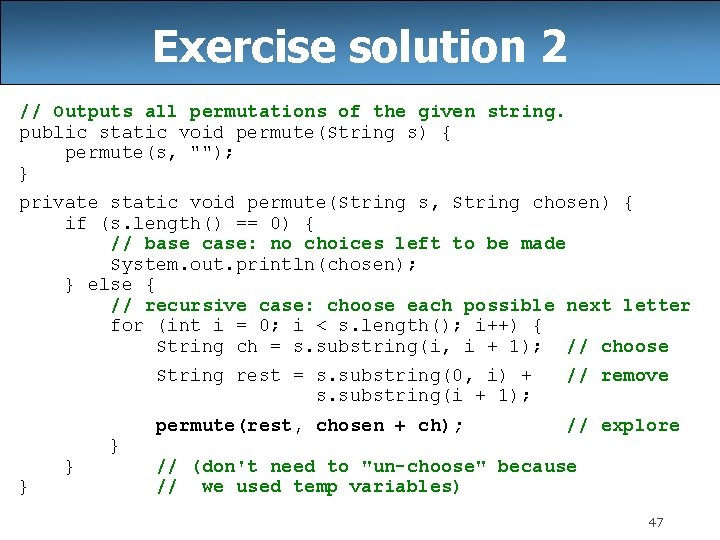Exercise solution 2 // Outputs all permutations of the given string. public static void permute(String s) { permute(s, ""); } private static void permute(String s, String chosen) { if (s. length() == 0) { // base case: no choices left to be made System. out. println(chosen); } else { // recursive case: choose each possible next letter for (int i = 0; i < s. length(); i++) { String ch = s. substring(i, i + 1); // choose } } } String rest = s. substring(0, i) + s. substring(i + 1); // remove permute(rest, chosen + ch); // explore // (don't need to "un-choose" because // we used temp variables) 47Exercise: Combinations • Write a method combinations that accepts a string s and an integer k as parameters and outputs all possible k -letter words that can be formed from unique letters in that string. The arrangements may be output in any order. – Example: combinations("GOOGLE", 3) outputs the sequence of lines at right. – To simplify the problem, you may assume that the string s contains at least k unique characters. EGL EGO ELG ELO EOG EOL GEO GLE GLO GOE GOL LEG LEO LGE LGO LOE LOG OEL OGE OGL OLE OLG 48Initial attempt public static void combinations(String s, int length) { combinations(s, "", length); } private static void combinations(String s, String chosen, int length) { if (length == 0) { System. out. println(chosen); // base case: no choices left } else { for (int i = 0; i < s. length(); i++) { String ch = s. substring(i, i + 1); if (!chosen. contains(ch)) { String rest = s. substring(0, i) + s. substring(i + 1); combinations(rest, chosen + ch, length - 1); } } – Problem: Prints same string multiple times. 49Exercise solution public static void combinations(String s, int length) { Set<String> all = new Tree. Set<String>(); combinations(s, "", all, length); for (String comb : all) { System. out. println(comb); } } private static void combinations(String s, String chosen, Set<String> all, int length) { if (length == 0) { all. add(chosen); // base case: no choices left } else { for (int i = 0; i < s. length(); i++) { String ch = s. substring(i, i + 1); if (!chosen. contains(ch)) { String rest = s. substring(0, i) + s. substring(i + 1); combinations(rest, chosen + ch, all, length - 1); } } 50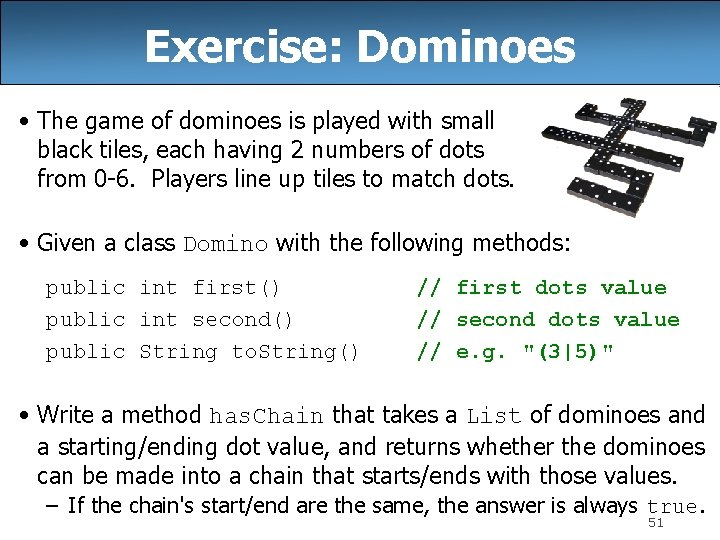Exercise: Dominoes • The game of dominoes is played with small black tiles, each having 2 numbers of dots from 0 -6. Players line up tiles to match dots. • Given a class Domino with the following methods: public int first() public int second() public String to. String() // first dots value // second dots value // e. g. "(3|5)" • Write a method has. Chain that takes a List of dominoes and a starting/ending dot value, and returns whether the dominoes can be made into a chain that starts/ends with those values. – If the chain's start/end are the same, the answer is always true. 51Domino chains • Suppose we have the following dominoes: • We can link them into a chain from 1 to 3 as follows: – Notice that the 3|5 domino had to be flipped. • We can "link" one domino into a "chain" from 6 to 2 as follows: 52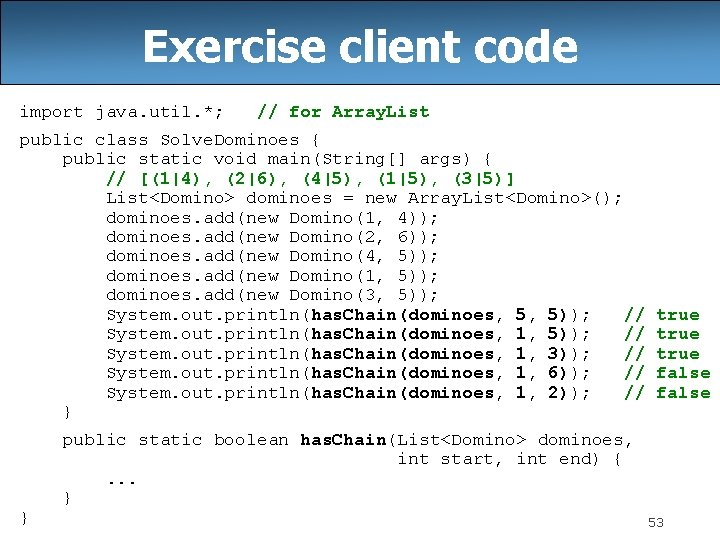Exercise client code import java. util. *; // for Array. List public class Solve. Dominoes { public static void main(String[] args) { // [(1|4), (2|6), (4|5), (1|5), (3|5)] List<Domino> dominoes = new Array. List<Domino>(); dominoes. add(new Domino(1, 4)); dominoes. add(new Domino(2, 6)); dominoes. add(new Domino(4, 5)); dominoes. add(new Domino(1, 5)); dominoes. add(new Domino(3, 5)); System. out. println(has. Chain(dominoes, 5, 5)); // System. out. println(has. Chain(dominoes, 1, 3)); // System. out. println(has. Chain(dominoes, 1, 6)); // System. out. println(has. Chain(dominoes, 1, 2)); // } } true false public static boolean has. Chain(List<Domino> dominoes, int start, int end) {. . . } 53Exercise solution public static boolean has. Chain(List<Domino> dominoes, int start, int end) { if (start == end) { return true; // base case } else { for (int i = 0; i < dominoes. size(); i++) { Domino d = dominoes. remove(i); // choose if (d. first() == start) { // explore if (has. Chain(dominoes, d. second(), end)) { return true; } } else if (d. second() == start) { if (has. Chain(dominoes, d. first(), end)) { return true; } } dominoes. add(i, d); // un-choose } return false; } } 54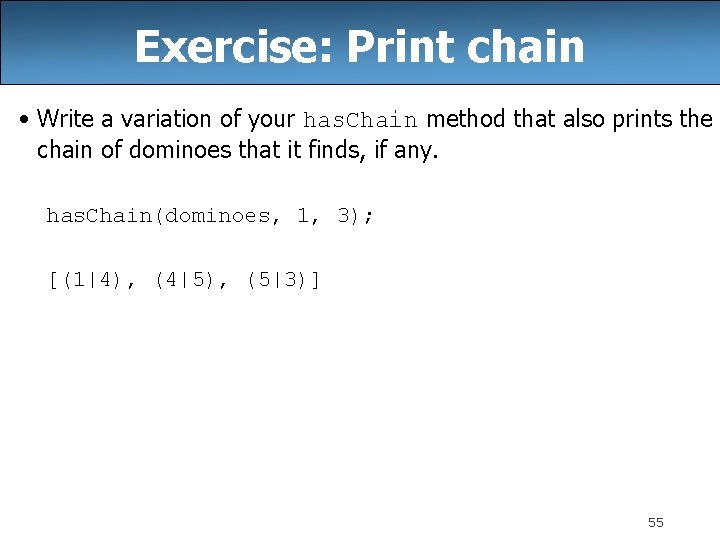Exercise: Print chain • Write a variation of your has. Chain method that also prints the chain of dominoes that it finds, if any. has. Chain(dominoes, 1, 3); [(1|4), (4|5), (5|3)] 55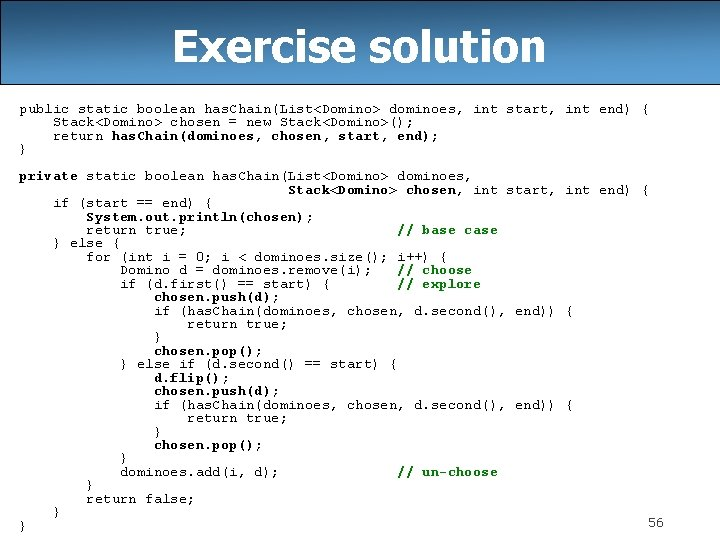Exercise solution public static boolean has. Chain(List<Domino> dominoes, int start, int end) { Stack<Domino> chosen = new Stack<Domino>(); return has. Chain(dominoes, chosen, start, end); } private static boolean has. Chain(List<Domino> dominoes, Stack<Domino> chosen, int start, int end) { if (start == end) { System. out. println(chosen); return true; // base case } else { for (int i = 0; i < dominoes. size(); i++) { Domino d = dominoes. remove(i); // choose if (d. first() == start) { // explore chosen. push(d); if (has. Chain(dominoes, chosen, d. second(), end)) { return true; } chosen. pop(); } else if (d. second() == start) { d. flip(); chosen. push(d); if (has. Chain(dominoes, chosen, d. second(), end)) { return true; } chosen. pop(); } dominoes. add(i, d); // un-choose } return false; } 56 }The "8 Queens" problem • Consider the problem of trying to place 8 queens on a chess board such that no queen can attack another queen. – What are the "choices"? Q Q – How do we "make" or "un-make" a choice? Q Q Q – How do we know when to stop? Q Q Q 57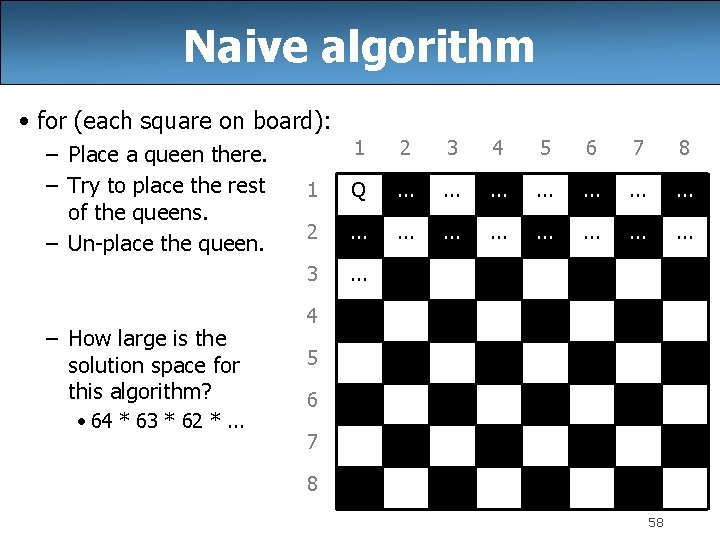Naive algorithm • for (each square on board): – Place a queen there. – Try to place the rest of the queens. – Un-place the queen. – How large is the solution space for this algorithm? • 64 * 63 * 62 *. . . 1 2 3 4 5 6 7 8 1 Q . . 2 . . . 3 . . . 4 5 6 7 8 58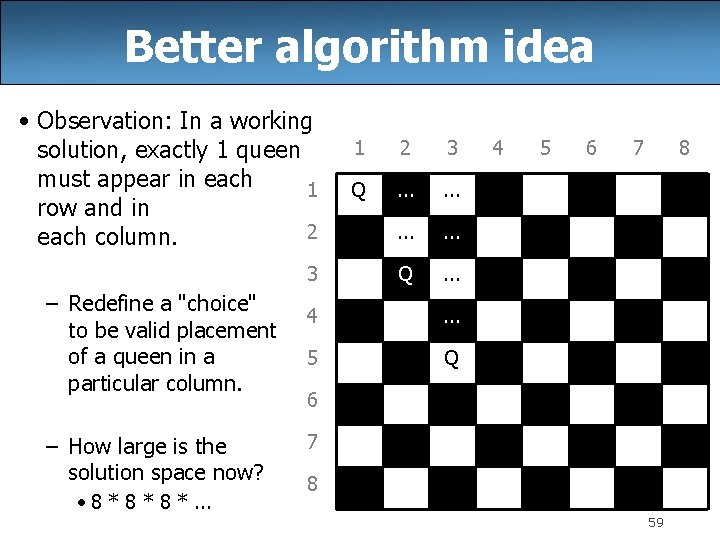Better algorithm idea • Observation: In a working solution, exactly 1 queen must appear in each 1 row and in 2 each column. 3 – Redefine a "choice" to be valid placement of a queen in a particular column. – How large is the solution space now? • 8 * 8 *. . . 1 2 3 Q . . . 4 . . . 5 Q 4 5 6 7 8 59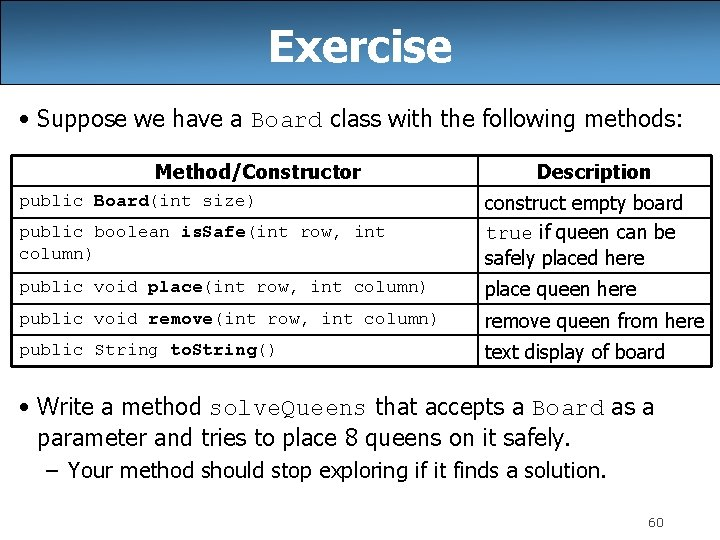Exercise • Suppose we have a Board class with the following methods: Method/Constructor Description public boolean is. Safe(int row, int column) construct empty board true if queen can be safely placed here public void place(int row, int column) place queen here public void remove(int row, int column) remove queen from here public String to. String() text display of board public Board(int size) • Write a method solve. Queens that accepts a Board as a parameter and tries to place 8 queens on it safely. – Your method should stop exploring if it finds a solution. 60Exercise solution // Searches for a solution to the 8 queens problem // with this board, reporting the first result found. public static void solve. Queens(Board board) { if (!explore(board, 1)) { System. out. println("No solution found. "); } else { System. out. println("One solution is as follows: "); System. out. println(board); } }. . . 61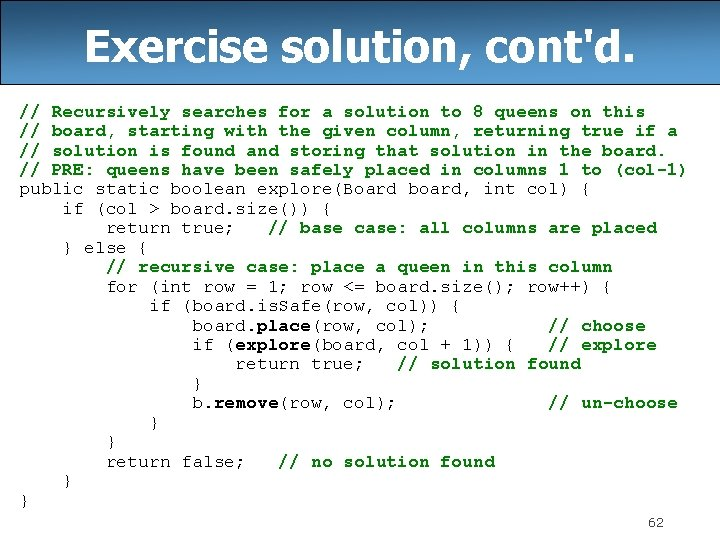Exercise solution, cont'd. // Recursively searches for a solution to 8 queens on this // board, starting with the given column, returning true if a // solution is found and storing that solution in the board. // PRE: queens have been safely placed in columns 1 to (col-1) public static boolean explore(Board board, int col) { if (col > board. size()) { return true; // base case: all columns are placed } else { // recursive case: place a queen in this column for (int row = 1; row <= board. size(); row++) { if (board. is. Safe(row, col)) { board. place(row, col); // choose if (explore(board, col + 1)) { // explore return true; // solution found } b. remove(row, col); // un-choose } } return false; // no solution found } } 62